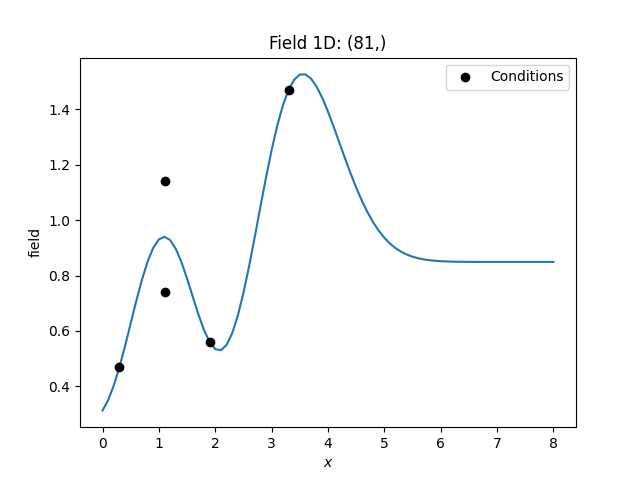Redundant data and pseudo-inverse

It can happen, that the kriging system gets numerically unstable. One reason could be, that the input data contains redundant conditioning points that hold different values.

To smoothly deal with such situations, you can use the pseudo inverse for the kriging matrix, which is enabled by default.

This will result in the average value for the redundant data.

Example

In the following we have two different values at the same location. The resulting kriging field will hold the average at this point.

import numpy as np

from gstools import Gaussian, krige

# condtions
cond_pos = [0.3, 1.9, 1.1, 3.3, 1.1]
cond_val = [0.47, 0.56, 0.74, 1.47, 1.14]
# resulting grid
gridx = np.linspace(0.0, 8.0, 81)
# spatial random field class
model = Gaussian(dim=1, var=0.5, len_scale=1)
krig = krige.Ordinary(model, cond_pos=cond_pos, cond_val=cond_val)
krig(gridx)
ax = krig.plot()
ax.scatter(cond_pos, cond_val, color="k", zorder=10, label="Conditions")
ax.legend()Total running time of the script: ( 0 minutes 0.140 seconds)

Gallery generated by Sphinx-Gallery